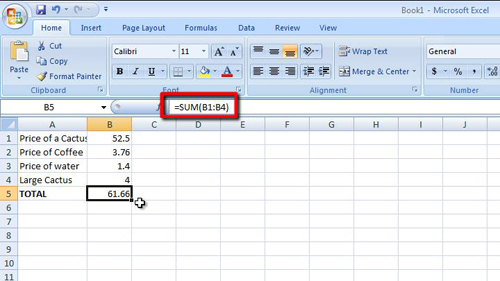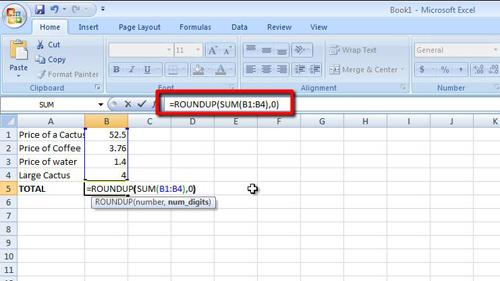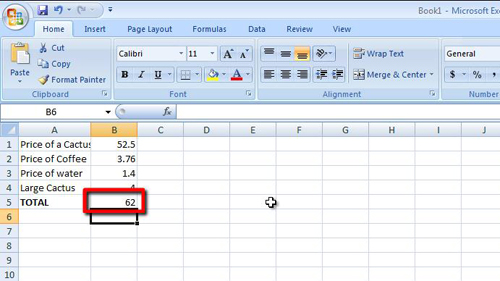## How to Make Excel 2007 Formulas Roundup

Often a formula will deliver a result that has a decimal place, this tutorial will show you how to make excel formulas roundup to prevent this issue.

Step # 1 – Using Just the SUM Formula

First off, load a spreadsheet that has some data in it. In this example we are going to be adding the price figures in column “B” of this sheet together. If we use the sum formula here we are left with “61.66”, we want this number to automatically round up to “62”.Step # 2 – Entering the SUM and ROUNDUP Formulas Together

Fortunately Excel comes with a formula that you can use in conjunction with others to make the result round up. This formula is known as the “ROUNDUP” formula. To use this first delete the SUM formula already entered, here it’s in cell “B5”. Now click on this cell and type, “=ROUNDUP(SUM(“. Now click and drag to highlight all of the numerical values you want to add together. Next type “),0)”.Step # 3 – Completing the Process

Press return and the formula will be applied. You will see here that the formula now rounds up the SUM result to “62”. That is how to make excel formulas roundup and the ROUNDUP formula can be used for many other formulas besides SUM.